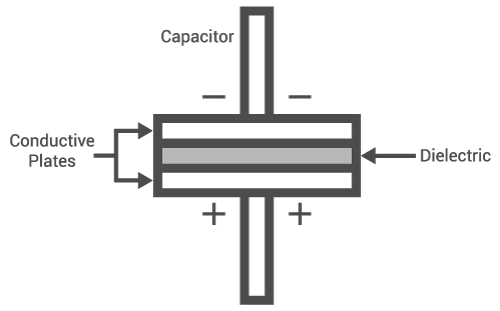# Capacitance, Reactance, and Admittance Calculator

## A calculator for solving the capacitance, reactance, and admittance of a capacitor.

(pF)
(Ω)
(S)

### Overview

This calculator is designed to compute for a capacitor's reactance and admittance given the capacitance value and the frequency. It can be also used the other way around, i.e., give the reactance value and it will give the capacitance. Note that the input capacitance must be in picofarads (pF) while the input frequency can be in Gigahertz (GHz) or Megahertz (MHz).### Equation

$$X_{C} = \frac{1}{2 \pi f C}$$

Where:

$$X_{C}$$ = capacitive reactance

$$f$$ = frequency

$$C$$ = capacitance

### Application

A capacitor's opposition to alternating current is called its reactance. This reactance is dependent on the frequency of the alternating current (see equation above). For low frequencies, the capacitor acts like a resistor with a very high resistance. In fact, at direct currents where the frequency is zero, the capacitor acts like an open switch. Conversely, the capacitor acts like a resistor with a very low resistance at very high frequencies. This characteristic of the capacitor makes it suitable for use in tons of applications, particularly in filters, phase shifters, and couplers.